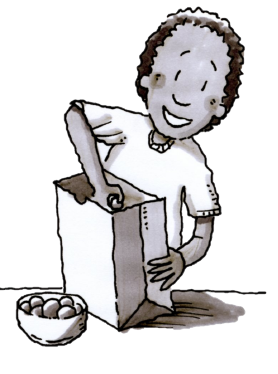Home > CC2MN > Chapter cc22 > Lesson cc22.2.3 > Problem2-64

2-64.John has a bag of marbles that contains $12$ red marbles, $20$ green marbles, and $17$ blue marbles. Homework Help ✎

1. If John pulls one marble out of the bag, what is the probability that it will not be red? That is, P(not red)?

Consider how many marbles are not red and how many total marbles are in his bag.
Then use these numbers to write the probability.

2. What is the probability that he will draw a purple marble? That is, P(purple)?

How many purple marbles are in his bag?

The probability is $0$. Make sure you know why and explain what a probability of $0$ means.